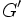# Nilpotent implies derived in Frattini

## Statement

For a Nilpotent group (?), the Commutator subgroup (?) (also called the derived subgroup) is contained inside the Frattini subgroup (?).

## Definitions used

### Commutator subgroup

Further information: commutator subgroup

The commutator subgroup of a group is defined as the intersection of all its Abelian-quotient subgroups, i.e. normal subgroups whose quotient group is Abelian.

### Frattini subgroup

Further information: Frattini subgroup

The Frattini subgroup of a group is defined as the intersection of all its maximal subgroups.

## Facts used

1. Nilpotent implies every maximal subgroup is normal: In a nilpotent group, every maximal subgroup is normal, and hence, a maximal normal subgroup
2. Any quotient of a nilpotent group is nilpotent. In particular, any quotient by a maximal normal subgroup is a simple nilpotent group
3. Any simple nilpotent group is Abelian

## Proof

Given: A nilpotent group$G$, with derived subgroup$G'$ and Frattini subgroup$\Phi(G)$

To prove:$G' \le \Phi(G)$

Proof: Since$G'$ is the intersection of all normal subgroups with Abelian quotients, and$\Phi(G)$ is the intersection of all maximal subgroups, it suffices to show that any maximal subgroup is normal with Abelian quotient.

By fact (1), every maximal subgroup is normal, and hence maximal normal. By fact (2), the quotient is a simple nilpotent group. By fact (3), the quotient is forced to be an Abelian group. Thus, every maximal subgroup is normal with Abelian quotient, and we are done.

## Generalizations

The result holds in a somewhat greater generality than nilpotent groups, if we can guarantee the following two things:

1. Any maximal subgroup is normal
2. Any simple quotient is Abelian

Thus, for instance, it holds for a solvable group satisfying the normalizer condition.